# Test: Network Equation (Mesh & Node) - 2

## 7 Questions MCQ Test Network Theory (Electric Circuits) | Test: Network Equation (Mesh & Node) - 2

Description
Attempt Test: Network Equation (Mesh & Node) - 2 | 7 questions in 30 minutes | Mock test for Electrical Engineering (EE) preparation | Free important questions MCQ to study Network Theory (Electric Circuits) for Electrical Engineering (EE) Exam | Download free PDF with solutions
QUESTION: 1

### i1 = ?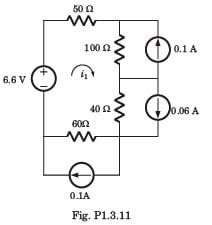Solution: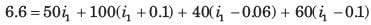i1 = 0.02 A

QUESTION: 2

### For the circuit of Fig. P1.3.38 the value of vs, that will result in v1 = 0, is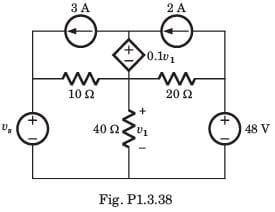Solution:

If v1 = 0, the dependent source is a short circuit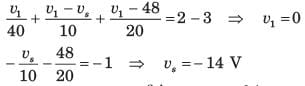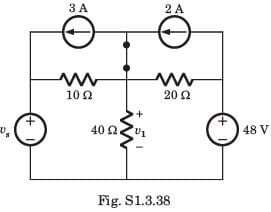QUESTION: 3

### i1, i2 = ?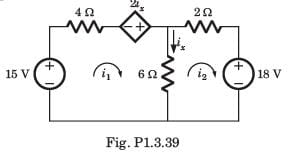Solution:

ix = i1 - i2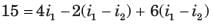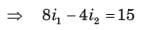....(i)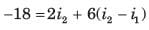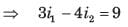...(ii)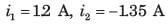QUESTION: 4

v1 = ?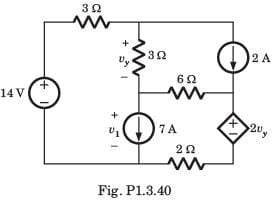Solution: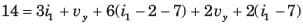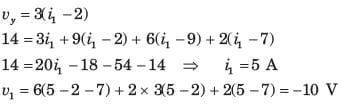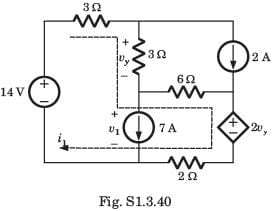QUESTION: 5

vx = ?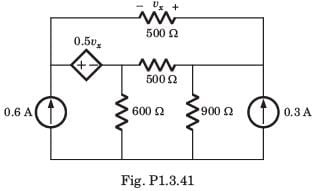Solution:

Let i1 and i2 be two loop current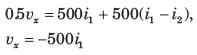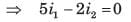...(i)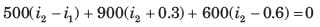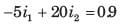...(ii)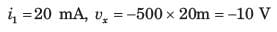QUESTION: 6

The power being dissipated in the 2Ω resistor in the circuit of Fig. P1.3.42 is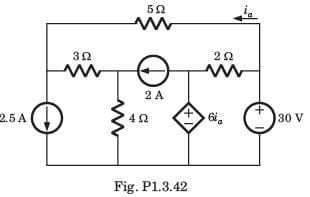Solution: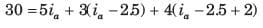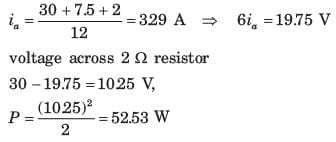QUESTION: 7

i1 = ?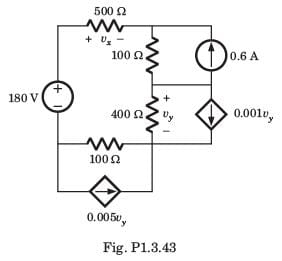Solution: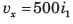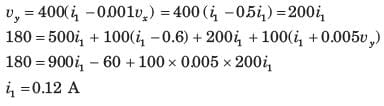Use Code STAYHOME200 and get INR 200 additional OFF Use Coupon Code